﻿ 基于MATLAB构建人口数学模型研究二胎开放对中国人口的影响 Constructing Population Mathematical Model Based on MATLAB to Study the Influence of the Second Birth Opening on Chinese Population

Vol.07 No.05(2018), Article ID:25044,19 pages
10.12677/AAM.2018.75066

Constructing Population Mathematical Model Based on MATLAB to Study the Influence of the Second Birth Opening on Chinese Population

Guangzheng Jing, Ruotong Wang, Fei Zhao, Juhe Sun

College of Electronic Information Engineering, Shenyang Aerospace University, Shenyang Liaoning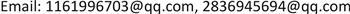Received: Apr. 26th, 2018; accepted: May 12th, 2018; published: May 24th, 2018ABSTRACT

In response to the current problems of aging in China, unbalanced labor supply and demand, and family pressure, our country has introduced a comprehensive two-child policy. This article is an analysis of the impact of the comprehensive two-child policy on the Chinese population. The population of the Chinese population from 2015 to 2030 is forecasted by improving the Leslie matrix population prediction algorithm, as well as the progressive deduction method and the queue element method based on the demographic factors and demographic principles of the population itself. Applying MATLAB and SPSS software, long-term and short-term labor problems, ageing issues, and dependency ratios after the implementation of the second-child policy are predicted. Comparing the data obtained with the existing data, it is concluded that the opening of the second child has a positive effect on China’s economic development, the alleviation of aging, and the increase in the number of laborers.

Keywords:Comprehensive Two-Child Policy, Population Growth, Improved Leslie Matrix, Queue Element Method Based on Demographic Factors and Demographic Principles, Aging, Labor Force1. 引言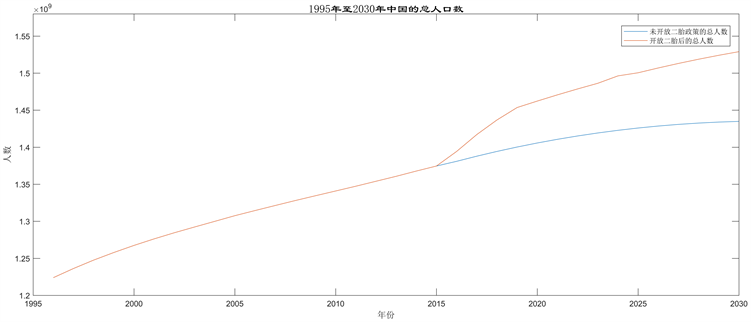注：图1中未开放全面二孩政策的情况下，以基于人口本身变动要素和人口学原理的队列要素法统一预测每年增加1700万新生儿。

Figure 1. Trends in total population from 1996 to 2030

2. 开放全面二孩政策后2015~2030年人口数学模型的建立

${Z}_{\mathrm{max}}=\theta ×{S}_{\mathrm{max}}$ (注：出生人口增量的上限) (1)

${Z}_{\mathrm{min}}=\theta ×{S}_{\mathrm{min}}$ (注：出生人口增量的下限) (2)

${Q}_{\mathrm{max}}=1700+{Z}_{\mathrm{max}}$ (注：总出生人口的上限) (3)

${Q}_{\mathrm{min}}=1700+{Z}_{\mathrm{min}}$ (注：总出生人口的下限) (4)

${Q}_{\text{mid}}=\frac{{Q}_{\mathrm{max}}+{Q}_{\mathrm{min}}}{2}$ (注：平均出生人口)(5)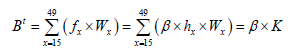(6)

${P}_{x+1}^{t}={P}_{x}^{t}×\left(1+{D}_{x}\right)$ (7)

${P}^{t}=\sum _{x=0}^{120}{P}_{x+1}^{t}$ (8)

${P}_{0}^{t+1}={B}^{t}+\left(1-{D}_{0}\right)$ (9)

$P={P}^{t}+{P}_{0}^{t+1}$ (10)

$\begin{array}{l}{P}_{A}={P}_{65+}/P\\ {R}_{d}=\left({P}_{0-18}+{P}_{65+}\right)/P\end{array}$ (11)Table 1. Forecast of birth and new born population from 2017 to 2020

${J}_{i1}={J}_{\left(i-1\right)1}×0.8×\left(1-{D}_{1}\right)+17000000$ (12)

${J}_{im}=\left({J}_{\left(i-1\right)\left(m-1\right)}×0.2+{J}_{\left(i-1\right)m}×0.8\right)×\left(1-{D}_{m}\right)$ (13)

${S}_{i}=\sum _{i=1}^{20}{J}_{im}$ (14)Table 3. Total segmental age population and mortality in 2015Table 4. Total number of people in each age group without full open two-child policy from 2015 to 2030

ContinuedTable 5. Implementation of comprehensive two-child policy 2020, 2025, 2030 population in all age groups

2) 开放全面二孩政策后2015~2065年人口数学模型的建立

$\left\{\begin{array}{l}{n}_{i}\left(t+1\right)=\sum _{i=1}^{m}{b}_{i}{n}_{i}\left(t\right)\\ {n}_{i+1}\left(t+1\right)={s}_{i}{n}_{i}\left(t\right),\text{\hspace{0.17em}}i=1,2,\cdots ,m-1\end{array}$ (15)

$L=\left[\begin{array}{ccccc}{b}_{1}& {b}_{2}& \cdots & {b}_{m-1}& {b}_{m}\\ {s}_{1}& 0& & & 0\\ 0& {s}_{2}& & & ⋮\\ & & \ddots & & \\ 0& & 0& {s}_{m-1}& 0\end{array}\right]$ (16)

$n\left(t+1\right)=Ln\left(t\right)$ (17)

$n\left(t\right)={L}^{t}n\left(0\right)$ (18)

1) ${s}_{i}>0,i=1,2\cdots ,m-1$

2) ${b}_{i}\ge 0,i=1,2\cdots ,m$ ，且至少一个 ${b}_{i}>0$

3) ${{b}^{\prime }}_{i}=\alpha {b}_{i}\left(i=0,1,2,\cdots ,90\right)$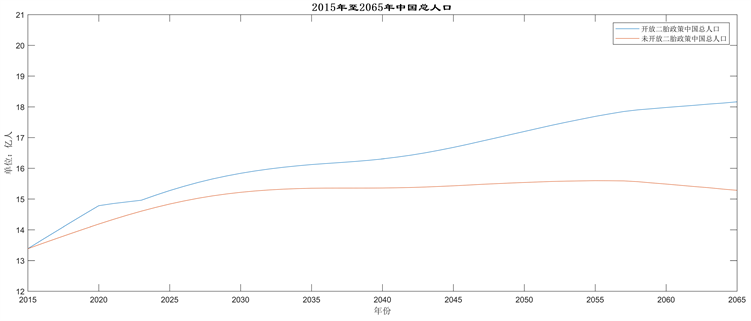Figure 2. Trends in total population from 2015 to 2065

3. 全面二孩政策对我国目前存在的社会问题的影响

3.1. 对我国劳动力供需问题的影响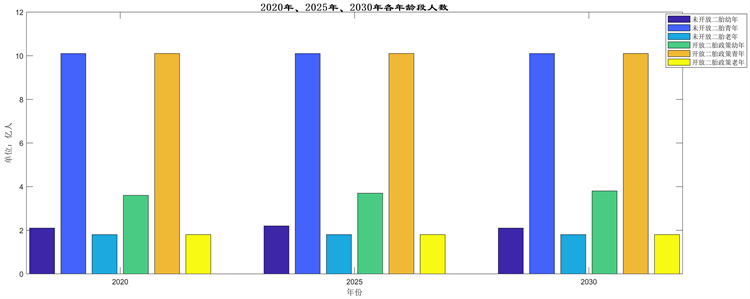Figure 3. 2020, 2025, 2030 young youth aged population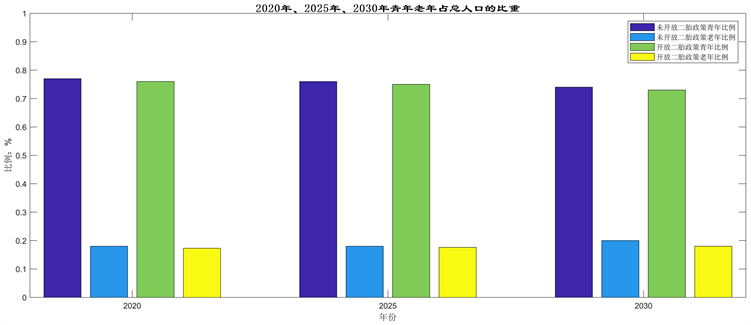Figure 4. Proportion of young people in the total population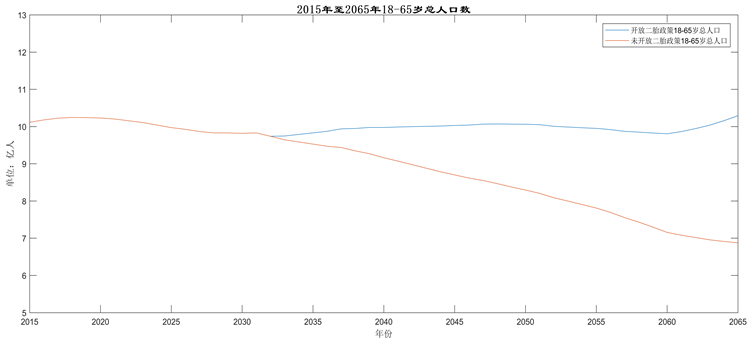Figure 5. Total population aged 18~65 from 2015 to 2065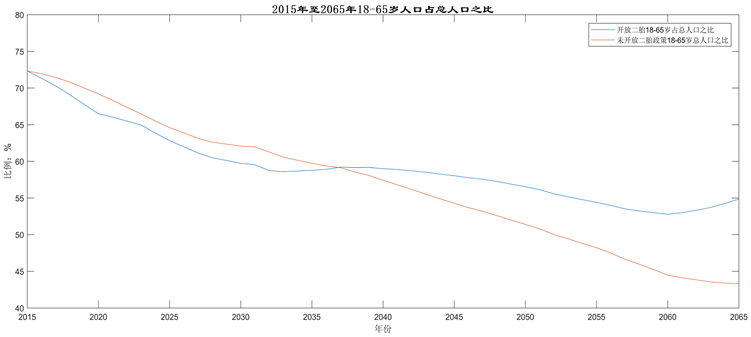Figure 6. Ratio of population aged 18~65 in total population from 2015 to 2065

3.2. 对人口老龄化的影响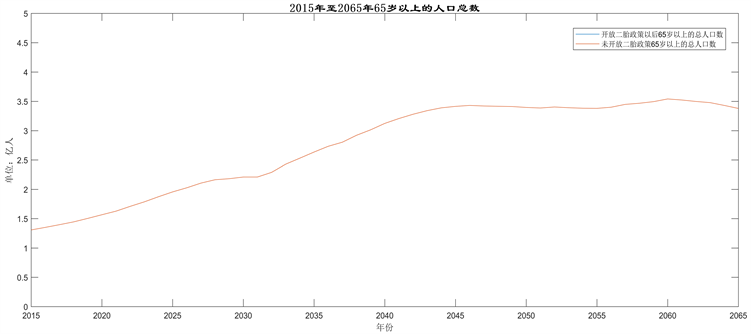Figure 7. Total population aged 65 and over from 2015 to 2065Figure 8. Ratio of population aged over 65 to total population from 2015 to 2065

3.3. 对抚养比的影响

$F=\frac{{L}_{i}+{Y}_{i}}{{R}_{i}}$ (19)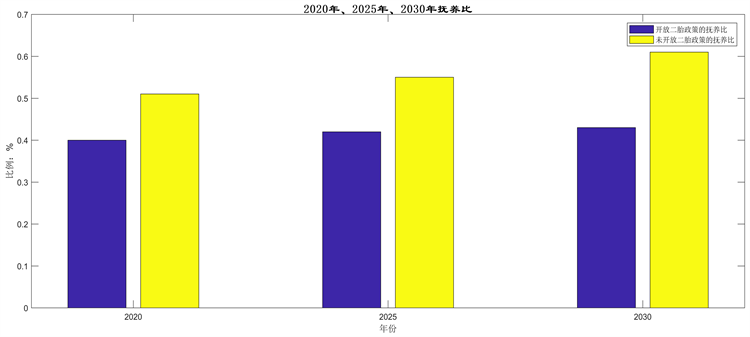Figure 9. Dependency ratio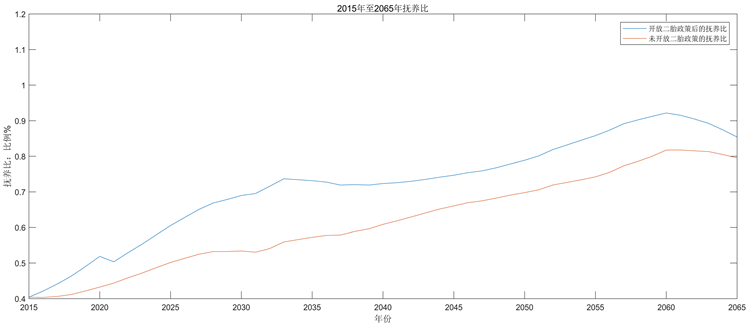Figure 10. Dependency ratios from 2015 to 2065

4. 开放二胎对我国经济与医疗的影响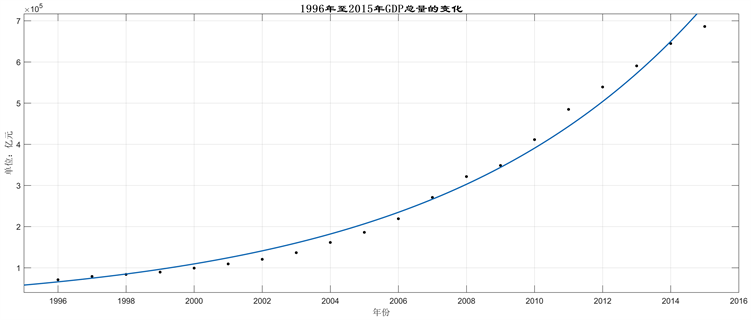Figure 11. Graph of changes in total GDP from 1996 to 2015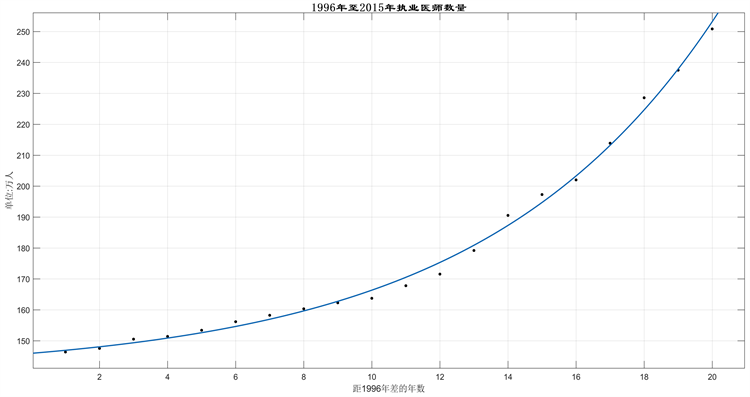Figure 12. Total number of licensed physicians from 1996 to 2015

5. 开放二胎对我国经济与医疗的影响

1) 使用了横向比较和纵向比较，横向比较用基于人口本身变动要素和人口学原理的队列要素法与改进型leslie矩阵模型，进行数值估计得比较，可以验证模型的准确性。全面开放二孩与未全面开放二孩比较，直观的反映开放二孩政策带来的总人口，劳动人口，老年人比重的变化，辩证对比，更易得出结论。

2) 模型简单易懂，可靠性良好，是两个模型预测出的2015至2030年的数据大致相同，可信度很高。

3) 模型在图像处理和显示上本文采用SPSS软件和MATLAB软件双重作图，拟合数据的变化趋势良好，使问题更加清晰，条理直观。

4) 数据的筛选大量使用中国统计局权威网站的数据，保证了数据真实有效，提升预测结论的。

6. 结论

1) 根据图8可以得出，在短时间内，全面开放二孩政策不会使65岁以上人口在总人口的比重中减小，只是相对减缓增长趋势，而且老龄化程度仍旧不断加深，所以，全面开放二孩，应作为一项长期坚持的国策才能使老龄化得到缓解，甚至出现扭转的局面。

2) 要坚持医疗卫生条件的发展，使医疗卫生水平不断得到改善。要坚持发展经济，保持经济的快速增长，才能在2050年实现我国在建国100周年，达到中等发达国家水平，实现中华民族伟大复兴实现“中国梦”。

3) 在短时间内新生儿数量，会出现“井喷式”爆发，全面开放二孩政策实施以后要注重基础教育和学前教育的发展，教育资源要跟幼年人口数量相匹配，大力发展我国教育事业，尤其是义务教育的力度，使我国的教育水平和力度能够满足社会需要。

4) 要大力增加就业岗位，鼓励民众自主创新创业，使新增劳动人口可以顺利就业。

Constructing Population Mathematical Model Based on MATLAB to Study the Influence of the Second Birth Opening on Chinese Population[J]. 应用数学进展, 2018, 07(05): 546-564. https://doi.org/10.12677/AAM.2018.75066

1. 1. 王开泳, 丁俊, 王甫园. 全面二孩政策对中国人口结构及区域人口空间格局的影响[J]. 地理科学进展, 2016, 35(11): 1305-1316.

2. 2. 中国国家统计局网站[Z/OL]. http://www.stats.gov.cn

3. 3. Guo, Z.G., Liu, J.T. and Song, J. Present Population Policy and the Family Structure [J]. 中国人口科学, 2002(1): 1-11.

4. 4. 百度文库. leslie人口增长模型[Z/OL].
https://wenku.baidu.com/view/0fe82e53c5da50e2534d7f0e.html

5. 5. 王文波. 数学建模及其基础知识详解[M]. 武汉: 武汉大学出版社, 2006.

6. 6. 张威. MATLAB基础与编程入门[M]. 第二版. 西安: 西安电子科技大学出版社, 2008.

$X\left(t\right)$ ：1996年后的第t年总人口数

${X}_{m}$ ：最大人口数

${X}_{0}$ ：1996年的人口数

r：人口增长率

${B}^{t}$ ：t年出生人口数

${f}_{x}$ ：分年龄段生育率

${W}_{x}$ ：分年龄育龄妇女数

${h}_{x}$ ：分年龄育龄妇女标准化生育率

$K$ ：标准化生育人口数

${S}_{\mathrm{max}}$ ：短期内潜在生育人群上限

${S}_{\mathrm{max}}$ ：短期内潜在生育人群上限

${Z}_{\mathrm{max}}$ ：出生人口增量上限

${Z}_{\mathrm{min}}$ ：出生人口增量下限

${Q}_{\mathrm{max}}$ ：出生人口总量上限

 ：出生人口总量下限

${Q}_{\text{mid}}$ ：出生总量均值

$\beta$ ：总和生育率

$\theta$ ：逐年释放率

${J}_{im}$ ：假设2015后的i年仍坚持计划生育，则第m段，年龄的人口数

${D}_{m}$ ：第m段，年龄的死亡率

${S}_{i}$ ：2015年后第i年的全国总人口数

m：将中国的人口分为m个年龄段，从0开始每1岁一组， $m=1,2,3,\cdots$

${n}_{i}\left(t\right),i=1,2,\cdots ,m$ ：在时间段t第i年龄组的人口总数

${b}_{i}\left(i=0,1,2,\cdots ,90\right)$ ：第i年龄组的生育率

${d}_{i}\left(i=0,1,2,\cdots ,90\right)$ ：第i年龄组的死亡率

${s}_{i}\left(i=0,1,2,\cdots ,90\right)$ ：第i年龄组的存活率

L：Leslie矩阵

$\lambda \left(x\right)$ ：距1996年的第x年职业医师总数

$\theta \left(x\right)$ ：第x年GDP总量

$\alpha$ ：调整系数

${{b}^{\prime }}_{i}$ ：调整后的第i年龄组的生育率

F：抚养比

${L}_{i}$ ：老年人数

${Y}_{i}$ ：幼年人数

${R}_{i}$ ：劳动力总人数